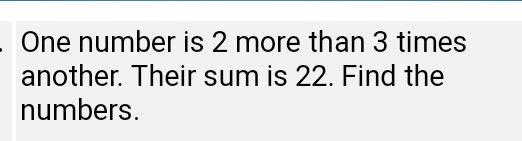# One number is 2 more than 3 times another their sum is 22 find the numbersOne number is 2 more than 3 times another. Their sum is 22. Find the numbers.

Let one of the number be X
As per the given data the another number is 2 more than 3 times
the another,
So the equation for another number be :- 2+ 3 X :- eq(1)
The sum of these two numbers is given as 22
In order to find out the numbers we can write the equations
as
(2+3 X) + X = 22:- eq(2)
Step by step the equation can be solved as shown below
3X + X = 22-2
4X = 20
X = 20/4
From the above result we get one of the number as 5 ,i.e X=5
substituting the value of x in eq(1) we get the another
number
2+3*5=17
So the two numbers are 5 & 17
17+5=22